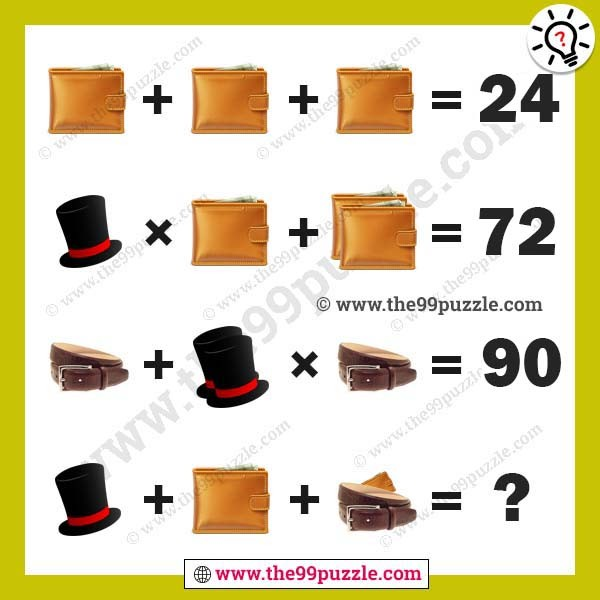# Wallet, cap, belt picture puzzles equation for genius – Puzz179

Wallet, cap, belt picture puzzles equation for genius. It is a simple math equation picture puzzle to test your brain. This is an easy viral math puzzle. However, if you are not clear with the BODMAS rule, you may get a different answer. Let us see if you can answer this math equation picture puzzle correctly and find the value of the missing number which replaces the question mark?###### Explanation:

Wallet + Wallet + Wallet = 24

Cap × Wallet + (Wallet+Wallet) = 72

Belt + (Cap+Cap) × Belt = 90

Cap + Wallet + (Belt+Wallet) = ?

8+8+8 = 24

7×8+(8+8) = 72

6+(7+7)×6 = 90

7+8+(6+8) = 29